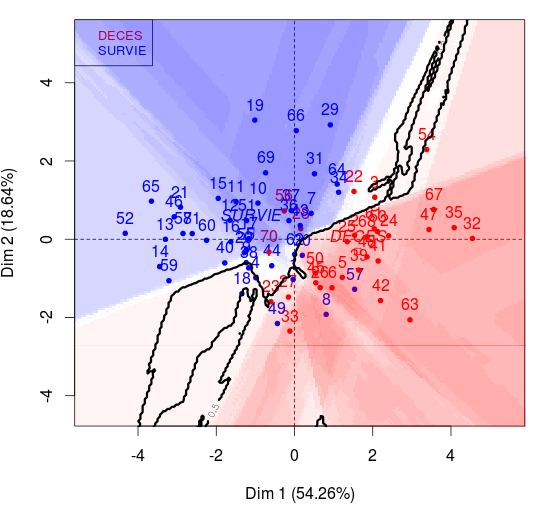# Growing Some Trees

· Big Data Zone · Interview
Save
4.84K Views

consider here the dataset used in a previous post , about visualising a classification (with more than 2 features),

``````> myocarde=read.table(
+ "http://freakonometrics.free.fr/saporta.csv",

the default classification tree is

``````> arbre = rpart(factor(prono)~.,data=myocarde)
> rpart.plot(arbre,type=4,extra=6)``````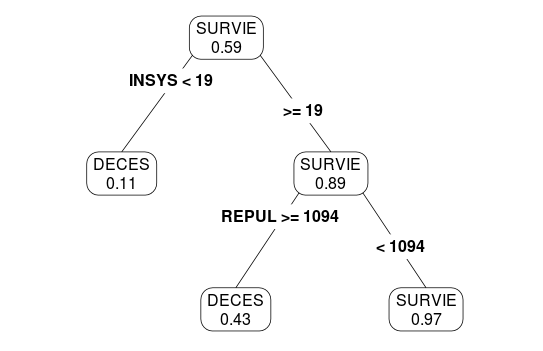we can change the options here, such as the minimum number of observations, per node

``````> arbre = rpart(factor(prono)~.,data=myocarde,
+       control=rpart.control(minsplit=10))
> rpart.plot(arbre,type=4,extra=6)``````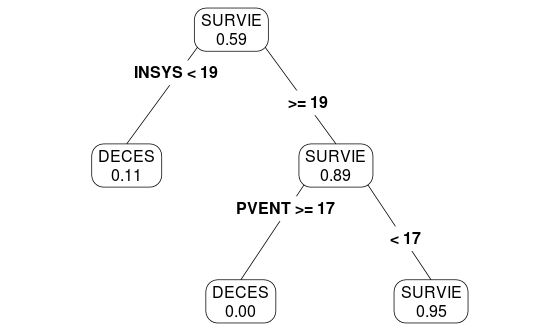or

``````> arbre = rpart(factor(prono)~.,data=myocarde,
+        control=rpart.control(minsplit=5))
> rpart.plot(arbre,type=4,extra=6)``````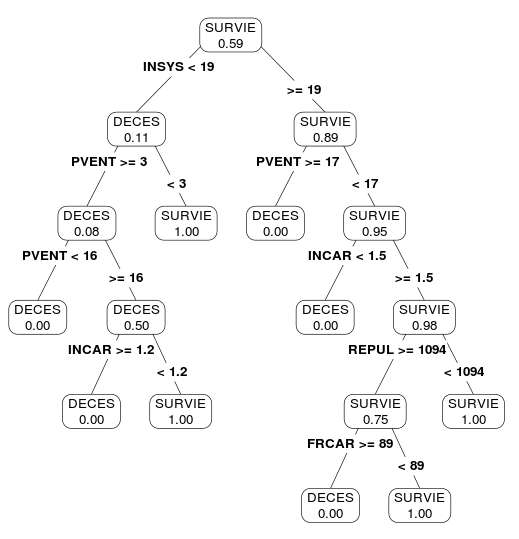to visualize that classification, use the following code (to get a projection on the first two components)

``````> library(factominer) # acp (sur les var continues)
> x = myocarde[,1:7]
> acp = pca(x,ncp=ncol(x))
> m = acp\$var\$coord
> minv = solve(m)
> m = apply(x,2,mean)
> s = apply(x,2,sd)
>
> arbre = rpart(factor(prono)~.,data=myocarde)
> pred2=function(d1,d2,mat,tree){
+   z=mat %*% c(d1,d2,rep(0,ncol(x)-2))
+   newd=data.frame(t(z*s+m))
+   names(newd)=names(x)
+   predict(tree,newdata=newd,
+           type="prob") }
> p=function(d1,d2) pred2(d1,d2,minv,arbre)

> outer <- function(x,y,fun) {
+   mat <- matrix(na, length(x), length(y))
+   for (i in seq_along(x)) {
+     for (j in seq_along(y))
+       mat[i,j]=fun(x[i],y[j])}
+   return(mat)}

> xgrid=seq(-5,5,length=251)
> ygrid=seq(-5,5,length=251)
> zgrid=outer(xgrid,ygrid,p)
> bluereds=c(
+   rgb(1,0,0,(10:0)/25),rgb(0,0,1,(0:10)/25))

> acp2=pca(myocarde,quali.sup=8,graph=true)
> plot(acp2, habillage = 8,col.hab=c("red","blue"))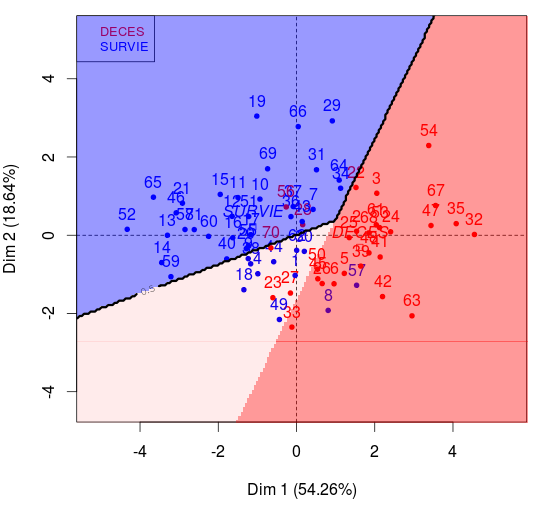it is also possible to consider the case where

``````> arbre = rpart(factor(prono)~.,data=myocarde,
+        control=rpart.control(minsplit=5))``````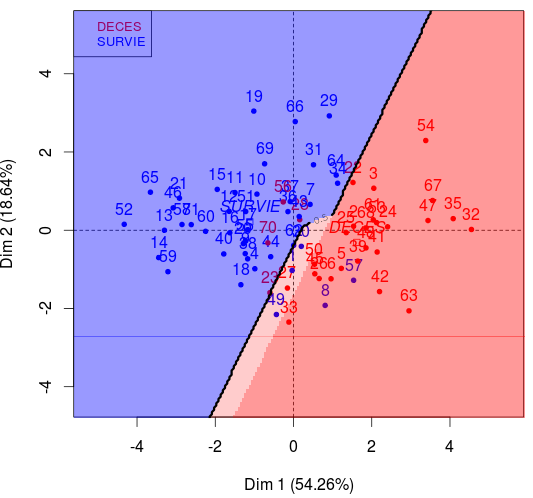finaly, one can also grow more trees, obtained by sampling. this is the idea of bagging : we boostrap our observations, we grow some trees, and then, we aggregate the predicted values. on the grid

``````> xgrid=seq(-5,5,length=201)
> ygrid=seq(-5,5,length=201)``````

the code is the following,

``````> z = matrix(0,201,201)
> for(i in 1:200){
+ indice = sample(1:nrow(myocarde),
+          size=nrow(myocarde),
+          replace=true)
+ echantillon=myocarde[indice,]
+ arbre_b = rpart(factor(prono)~.,
+   data=echantillon)
+ p2 = function(d1,d2) pred2(d1,d2, minv,arbre_b)
+ zgrid2_b = outer(xgrid,ygrid,p2)
+ z = z+zgrid2_b }
> zgrid = z/200``````

to visualize it, use

``````> plot(acp2, habillage = 8,
+ col.hab=c("red","blue"))
+ col=bluereds)``````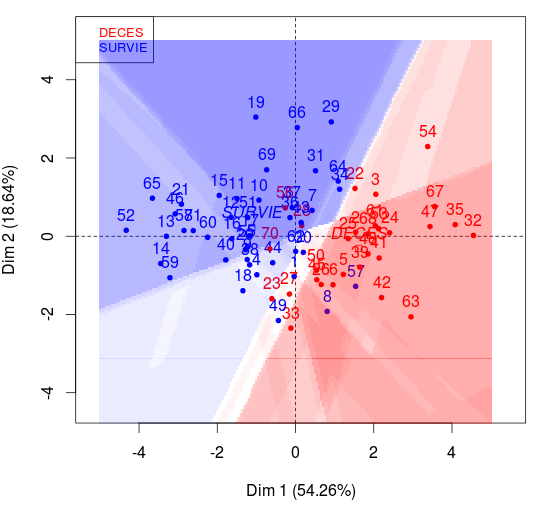``````> contour(xgrid,ygrid,zgrid,add=true,
+ levels=.5,lwd=3)``````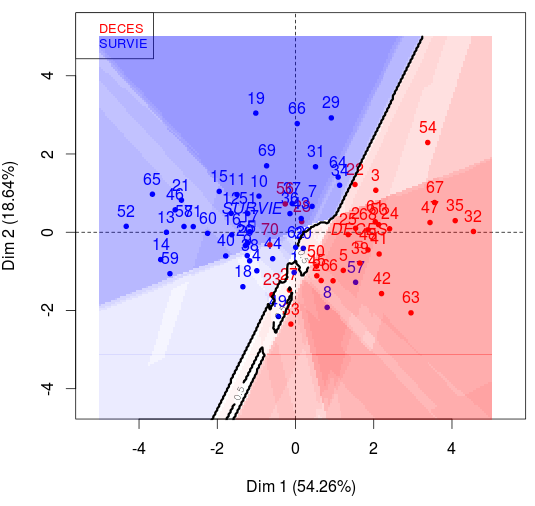last, but not least, it is possible to use some random forrest algorithm. the method combines breiman’s bagging idea (mentioned previously) and the random selection of features.

``````> library(randomforest)
> foret = randomforest(factor(prono)~.,
+          data=myocarde)
> pf=function(d1,d2) pred2(d1,d2,minv,foret)
> zgridf=outer(xgrid,ygrid,pf)

> acp2=pca(myocarde,quali.sup=8,graph=true)
> plot(acp2, habillage = 8,col.hab=c("red","blue"))
+ col=bluereds)
> contour(xgrid,ygrid,zgridf,